## Truncated OctahedronAn Archimedean Solid, also known as the Mecon, whose Dual Polyhedron is the Tetrakis Hexahedron. It is also Uniform Polyhedronand has Schläfli Symbol tand Wythoff Symbol. The faces of the truncated octahedron are. The truncated octahedron has the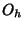Octahedral Group of symmetries.The solid can be formed from an Octahedron via Truncation by removing six Square Pyramids, each with edge slant heightand height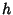, whereis the side length of the original Octahedron. From the above diagram, the height and base area of the Square Pyramid are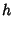(1)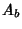(2)

The Volume of the truncated octahedron is then given by the Volume of the Octahedron(3)

minus six times the volume of the Square Pyramid,(4)

The truncated octahedron is a Space-Filling Polyhedron. The Inradius, Midradius, and Circumradius forare(5)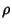(6)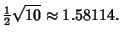(7)